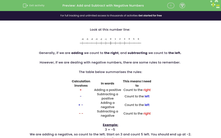# Add and Subtract with Negative Numbers

In this worksheet, students will look at addition and subtraction involving negative numbers.Key stage:  KS 3

Curriculum topic:   Number

Curriculum subtopic:   Use Four Operations for All Numbers

Difficulty level:#### Worksheet Overview

Look at this number line:Generally, if we are adding we count to the right, and subtracting we count to the left.

However, if we are dealing with negative numbers, there are some rules to remember.

The table below summarises the rules:

 Calculation involves In words This means I need to + Adding a positive Count to the right - Subtracting a positive Count to the left + - Adding a negative Count to the left - - Subtracting a negative Count to the right

Example:

3 + -5

We are adding a negative, so count to the left. Start on 3 and count 5 left. You should end up at -2.

so 3 + -5 = -2

Example:

6 - -7

We are subtracting a negative, so count to the right. Start on 6 and count 7 right. You should end up at 13

6 - -7 = 13It can help if you know these rules for operation signs that appear next to each other:

and +

- and +

+ and - = -

- and + = -

Let's practise this now in the questions that follow.

### What is EdPlace?

We're your National Curriculum aligned online education content provider helping each child succeed in English, maths and science from year 1 to GCSE. With an EdPlace account you’ll be able to track and measure progress, helping each child achieve their best. We build confidence and attainment by personalising each child’s learning at a level that suits them.

Get started